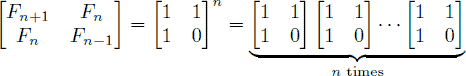# Fibonacci

Time Limit: 1000MS

Memory Limit: 65536K

[显示标签]

## Description

In the Fibonacci integer sequence, F0 = 0, F1 = 1, and Fn = Fn − 1 + Fn − 2 for n ≥ 2. For example, the first ten terms of the Fibonacci sequence are:0, 1, 1, 2, 3, 5, 8, 13, 21, 34, …An alternative formula for the Fibonacci sequence is.Given an integer n, your goal is to compute the last 4 digits of Fn.

## Input

The input test file will contain multiple test cases. Each test case consists of a single line containing n (where 0 ≤ n ≤ 1,000,000,000). The end-of-file is denoted by a single line containing the number −1.

## Output

For each test case, print the last four digits of Fn. If the last four digits of Fn are all zeros, print ‘0’; otherwise, omit any leading zeros (i.e., print Fn mod 10000).

## Sample Input

0
9
999999999
1000000000
-1

## Sample Output

0
34
626
6875

## Hint

As a reminder, matrix multiplication is associative, and the product of two 2 × 2 matrices is given by.

Also, note that raising any 2 × 2 matrix to the 0th power gives the identity matrix:.

## Source

Stanford Local 2006